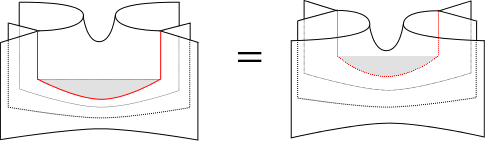## Data

• Title: The $$\mathfrak{sl}_3$$ web algebra
• Authors: Marco Mackaay, Weiwei Pan and Daniel Tubbenhauer
• Status: Math. Z. 277-1-2 (2014), 401-479. Last update: Sat, 10 Mar 2018 12:15:16 GMT
• ArXiv version = 0.99 published version
• LaTex Beamer presentation: Slides1

## Abstract

In this paper we use Kuperberg's $$\mathfrak{sl}_3$$ webs and Khovanov's $$\mathfrak{sl}_3$$ foams to define a new algebra $$K_S$$, which we call the $$\mathfrak{sl}_3$$ web algebra. It is the $$\mathfrak{sl}_3$$ analogue of Khovanov's arc algebra $$H(n)$$. We prove that $$K_S$$ is a graded symmetric Frobenius algebra. Furthermore, we categorify an instance of quantum skew Howe duality, which allows us to prove that $$K_S$$ is Morita equivalent to a certain, cyclotomic KLR-algebra. This allows us to determine the Grothendieck group of $$K_S$$, to show that its center is isomorphic to the cohomology ring of a certain Spaltenstein variety, and to prove that $$K_S$$ is a graded cellular algebra.

## A few extra words

The main idea of the $$\mathfrak{sl}_3$$ web algebra is simple, i.e. given four webs $$w_1,w_2,w_3$$ and $$w_4$$, which are the same at the boundary, one can define two new webs $$w_1w_2$$ and $$w_3w_4$$ by rotating $$w_1$$ and $$w_3$$ around the x-axis, s witching their orientation and glue them on top of $$w_2$$ and $$w_4$$. Then a multiplication $$m(f(w_1w_2),f(w_3w_4))$$ of foams with the corresponding webs as boundary can be defined as follows:

• If $$w_2$$ and $$w_3$$ are different, then, by convention, the multiplication is zero.
• If $$w_2=w_3$$, then glue the foams together via a multiplication foam.
• The multiplication foam is made of saddles (arc-split), unzips (Y-split) and square removals (H-split). The picture shows a saddle and an unzip.
• The picture below is one part of the proof that the multiplication does not depend on the isotopy type. Note that the part of the foams corresponding to $$w_1$$ and $$w_4$$ is not shown, since it will be the identity.
• Note that one can speak of a categorification, i.e. on web level the multiplication is trivial (erase the middle and glue top and bottom), but on foam level lots of interesting mathematics is happening.